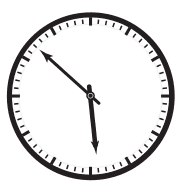### H - Hours and Minutes

##### Languages: C, C++, Java, JavaScript, ... (details)Heidi has a discrete analog clock in the shape of a circle, as the one in the figure. Two hands rotate around the center of the circle, indicating hours and minutes. The clock has $60$ marks placed around its perimeter, with the distance between consecutive marks being constant. The minute hand moves from its current mark to the next exactly once every minute. The hour hand moves from its current mark to the next exactly once every $12$ minutes, so it advances five marks each hour. We consider that both hands move discretely and instantly, which means they are always positioned exactly over one of the marks and never in between marks.

At midnight both hands reach simultaneously the top mark, which indicates zero hours and zero minutes. After exactly $12$ hours or $720$ minutes, both hands reach the same position again, and this process is repeated over and over again. Note that when the minute hand moves, the hour hand may not move; however, when the hour hand moves, the minute hand also moves. Heidi likes geometry, and she likes to measure the minimum angle between the two hands of the clock at different times of the day. She has been writing some measures down, but after several years and a long list, she noticed that some angles were repeated while some others never appeared.

For instance, Heidi’s list indicates that both at three o’clock and at nine o’clock the minimum angle between the two hands is $90$ degrees, while an angle of $65$ degrees does not appear in the list. Heidi decided to check, for any integer number A between 0 and 180, if there exists at least one time of the day such that the minimum angle between the two hands of the clock is exactly $A$ degrees. Help her with a program that answers this question.

#### Input

Each test case is described using one line. The line contains an integer $A$ representing the angle to be checked $(0 \leq A \leq 180)$.

#### Output

For each test case output a line containing a character. If there exists at least one time of the day such that the minimum angle between the two hands of the clock is exactly $A$ degrees, then write the uppercase letter “Y”. Otherwise write the uppercase letter “N”.

#### Sample test(s)

Input
90 65 66 67 128 0 180
Output
Y N Y N N Y Y